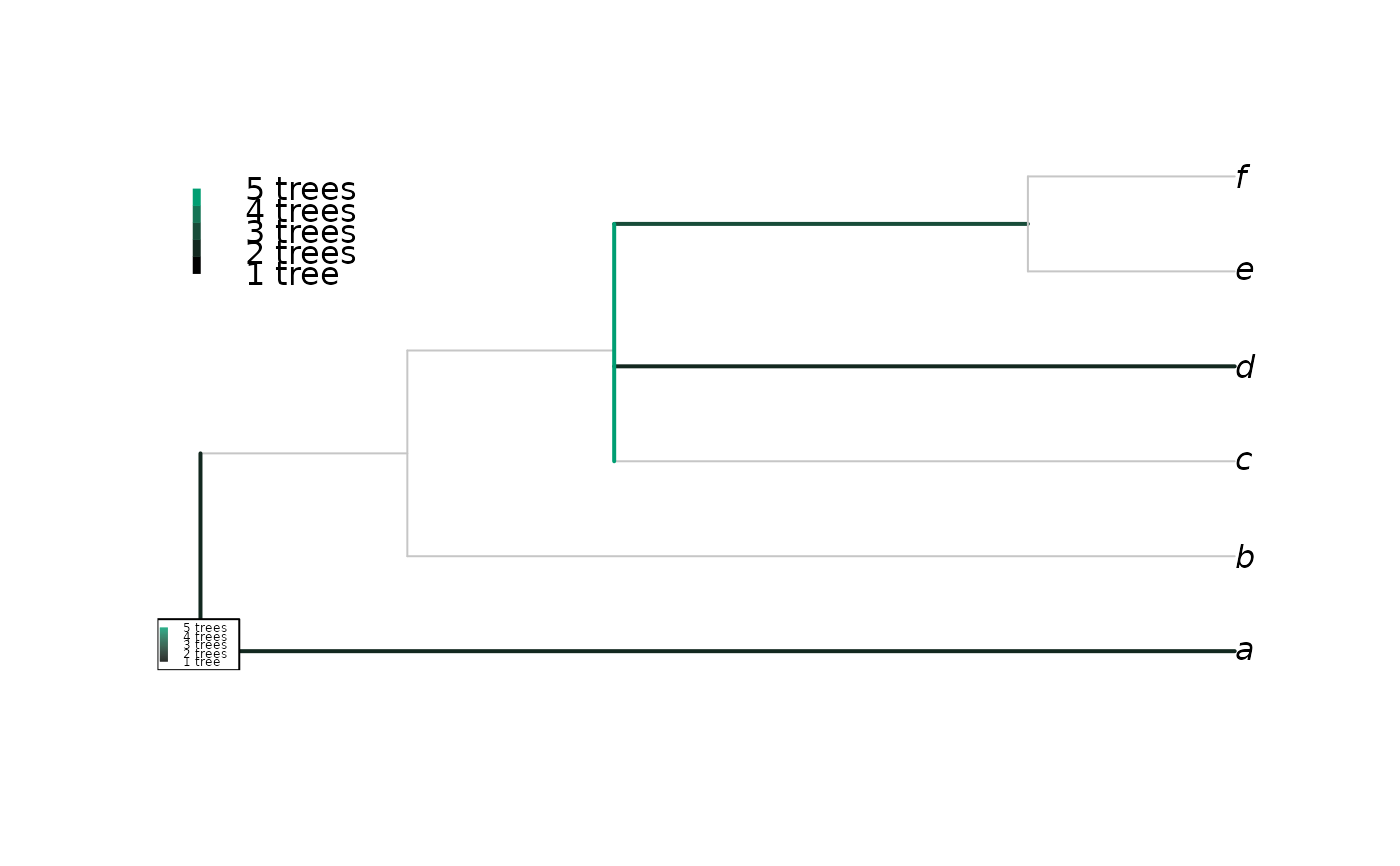Plots a consensus of trees with a rogue taxon omitted, with edges coloured according to the proportion of trees in which the taxon attaches to that edge, after Klopfstein and Spasojevic (2019) .

RoguePlot(
trees,
tip,
p = 1,
plot = TRUE,
Palette = colorRampPalette(c(par("fg"), "#009E73"), space = "Lab"),
nullCol = rgb(colorRamp(unlist(par(c("fg", "bg"))), space = "Lab")(0.8)/255),
edgeLength = NULL,
thin = par("lwd"),
fat = thin + 1L,
outgroupTips,
...
)

## Arguments

trees

List or multiPhylo object containing phylogenetic trees of class phylo to be summarized.

tip

Numeric or character identifying rogue leaf, in format accepted by DropTip().

p

A numeric value between 0.5 and 1 giving the proportion for a clade to be represented in the consensus tree (see Consensus()).

plot

Logical specifying whether to plot the tree.

Palette

Function that takes a parameter n and generates a colour palette with n entries.

nullCol

Colour to paint regions of the tree on which the rogue is never found.

edgeLength

Numeric specifying edge lengths of consensus tree; NULL aligns tips by scaling edges proportional to clade size; 1 sets all edges to unit length.

thin, fat

Numeric specifying width to plot edges if the rogue tip never / sometimes does attach to them.

outgroupTips

Vector of type character, integer or logical, specifying the names or indices of the tips to include in the outgroup. If outgroupTips is a of type character, and a tree contains multiple tips with a matching label, the first will be used.

...

Additional parameters to plot.phylo().

## Value

RoguePlot() returns a list whose elements are:

• cons: The reduced consensus tree, in preorder;

• onEdge: a vector of integers specifying the number of trees in trees in which the rogue leaf is attached to each edge in turn of the consensus tree;

• atNode: a vector of integers specifying the number of trees in trees in which the rogue leaf is attached to an edge collapsed into each node of the consensus tree.

## Details

Rogue taxa can be identified using the package Rogue (Smith 2022) .

Other consensus tree functions: ConsensusWithout(), Consensus()

## Author

Martin R. Smith (martin.smith@durham.ac.uk)

## Examples

trees <- list(read.tree(text = "(a, (b, (c, (rogue, (d, (e, f))))));"),
read.tree(text = "(a, (b, (c, (rogue, (d, (e, f))))));"),
read.tree(text = "(a, (b, (c, (rogue, (d, (e, f))))));"),
read.tree(text = "(a, (b, (c, (rogue, (d, (e, f))))));"),
read.tree(text = "(rogue, (a, (b, (c, (d, (e, f))))));"),
read.tree(text = "((rogue, a), (b, (c, (d, (e, f)))));"),
read.tree(text = "(a, (b, ((c, d), (rogue, (e, f)))));"),
read.tree(text = "(a, (b, ((c, (rogue, d)), (e, f))));"),
read.tree(text = "(a, (b, (c, (d, (rogue, (e, f))))));"))
RoguePlot(trees, "rogue")#> $cons #> #> Phylogenetic tree with 6 tips and 4 internal nodes. #> #> Tip labels: #> a, b, c, d, e, f #> #> Rooted; no branch lengths. #> #>$onEdge
#>  1 0 0 0 0 1 2 0 0
#>
#> \$atNode
#>  1 0 4 0
#>